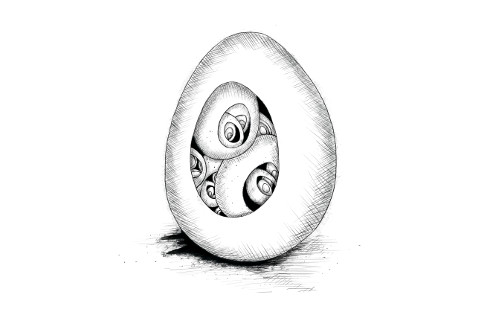## 十二、项目：编程语言

Hal Abelson 和 Gerald Sussman，《计算机程序的构造和解释》## 解析

``````do(define(x, 10),
if(>(x, 5),
print("large"),
print("small")))
``````

Egg 语言的一致性体现在：JavaScript 中的所有运算符（比如`>`）在 Egg 中都是绑定，但是可以像其他函数一样调用。由于语法中没有语句块的概念，因此我们需要使用`do`结构来表示多个表达式的序列。

``````{
type: "apply",
operator: {type: "word", name: ">"},
args: [
{type: "word", name: "x"},
{type: "value", value: 5}
]
}
````````````function parseExpression(program) {
program = skipSpace(program);
let match, expr;
if (match = /^"([^"]*)"/.exec(program)) {
expr = {type: "value", value: match};
} else if (match = /^\d+\b/.exec(program)) {
expr = {type: "value", value: Number(match)};
} else if (match = /^[^\s(),#"]+/.exec(program)) {
expr = {type: "word", name: match};
} else {
throw new SyntaxError("Unexpected syntax: " + program);
}

return parseApply(expr, program.slice(match.length));
}

function skipSpace(string) {
let first = string.search(/\S/);
if (first == -1) return "";
return string.slice(first);
}
``````

``````function parseApply(expr, program) {
program = skipSpace(program);
if (program != "(") {
return {expr: expr, rest: program};
}

program = skipSpace(program.slice(1));
expr = {type: "apply", operator: expr, args: []};
while (program != ")") {
let arg = parseExpression(program);
expr.args.push(arg.expr);
program = skipSpace(arg.rest);
if (program == ",") {
program = skipSpace(program.slice(1));
} else if (program != ")") {
throw new SyntaxError("Expected ',' or ')'");
}
}
return parseApply(expr, program.slice(1));
}
``````

``````function parse(program) {
let {expr, rest} = parseExpression(program);
if (skipSpace(rest).length > 0) {
throw new SyntaxError("Unexpected text after program");
}
return expr;
}

console.log(parse("+(a, 10)"));
// → {type: "apply",
//    operator: {type: "word", name: "+"},
//    args: [{type: "word", name: "a"},
//           {type: "value", value: 10}]}
``````

## 求值器（evaluator）

``````const specialForms = Object.create(null);

function evaluate(expr, scope) {
if (expr.type == "value") {
return expr.value;
} else if (expr.type == "word") {
if (expr.name in scope) {
return scope[expr.name];
} else {
throw new ReferenceError(
`Undefined binding: \${expr.name}`);
}
} else if (expr.type == "apply") {
let {operator, args} = expr;
if (operator.type == "word" &&
operator.name in specialForms) {
return specialForms[operator.name](expr.args, scope);
} else {
let op = evaluate(operator, scope);
if (typeof op == "function") {
return op(...args.map(arg => evaluate(arg, scope)));
} else {
throw new TypeError("Applying a non-function.");
}
}
}
}
``````

`evaluate`的递归结构类似于解析器的结构。两者都反映了语言自身的结构。我们也可以将解析器和求值器集成到一起，在解析的同时求解表达式，但将其分离为两个阶段使得程序更易于理解。

## 特殊形式

`specialForms`对象用于定义 Egg 中的特殊语法。该对象将单词和求解这种形式的函数关联起来。目前该对象为空，现在让我们添加`if`

``````specialForms.if = (args, scope) => {
if (args.length != 3) {
throw new SyntaxError("Wrong number of args to if");
} else if (evaluate(args, scope) !== false) {
return evaluate(args, scope);
} else {
return evaluate(args, scope);
}
};
``````

Egg 的`if`语句需要三个参数。Egg 会求解第一个参数，若结果不是`false`，则求解第二个参数，否则求解第三个参数。相较于 JavaScript 中的`if`语句，Egg 的`if`形式更类似于 JavaScript 中的`?:`运算符。这是一条表达式，而非语句，它会产生一个值，即第二个或第三个参数的结果。

Egg 和 JavaScript 在处理条件值时也有些差异。Egg 不会将 0 或空字符串作为假，只有当值确实为`false`时，测试结果才为假。

``````specialForms.while = (args, scope) => {
if (args.length != 2) {
throw new SyntaxError("Wrong number of args to while");
}
while (evaluate(args, scope) !== false) {
evaluate(args, scope);
}

// Since undefined does not exist in Egg, we return false,
// for lack of a meaningful result.
return false;
};
``````

``````specialForms.do = (args, scope) => {
let value = false;
for (let arg of args) {
value = evaluate(arg, scope);
}
return value;
};
``````

``````specialForms.define = (args, scope) => {
if (args.length != 2 || args.type != "word") {
throw new SyntaxError("Incorrect use of define");
}
let value = evaluate(args, scope);
scope[args.name] = value;
return value;
};
``````

## 环境

`evaluate`所接受的作用域是一个对象，它的名称对应绑定名称，它的值对应这些绑定所绑定的值。 我们定义一个对象来表示全局作用域。

``````const topScope = Object.create(null);

topScope.true = true;
topScope.false = false;
``````

``````let prog = parse(`if(true, false, true)`);
console.log(evaluate(prog, topScope));
// → false
``````

``````for (let op of ["+", "-", "*", "/", "==", "<", ">"]) {
topScope[op] = Function("a, b", `return a \${op} b;`);
}
``````

``````topScope.print = value => {
console.log(value);
return value;
};
``````

``````function run(program) {
return evaluate(parse(program), Object.create(topScope));
}
``````

``````run(`
do(define(total, 0),
define(count, 1),
while(<(count, 11),
do(define(total, +(total, count)),
define(count, +(count, 1)))),
print(total))
`);
// → 55
``````

## 函数

``````specialForms.fun = (args, scope) => {
if (!args.length) {
throw new SyntaxError("Functions need a body");
}
let body = args[args.length - 1];
let params = args.slice(0, args.length - 1).map(expr => {
if (expr.type != "word") {
throw new SyntaxError("Parameter names must be words");
}
return expr.name;
});

return function() {
if (arguments.length != params.length) {
throw new TypeError("Wrong number of arguments");
}
let localScope = Object.create(scope);
for (let i = 0; i < arguments.length; i++) {
localScope[params[i]] = arguments[i];
}
return evaluate(body, localScope);
};
};
``````

Egg 中的函数可以获得它们自己的局部作用域。 `fun`形式产生的函数创建这个局部作用域，并将参数绑定添加到它。 然后求解此范围内的函数体并返回结果。

``````run(`
do(define(plusOne, fun(a, +(a, 1))),
print(plusOne(10)))
`);
// → 11

run(`
do(define(pow, fun(base, exp,
if(==(exp, 0),
1,
*(base, pow(base, -(exp, 1)))))),
print(pow(2, 10)))
`);
// → 1024
``````

## 站在别人的肩膀上

``````behavior walk
perform when
actions
move left-foot
move right-foot

behavior attack
perform when
Godzilla in-view
actions
fire laser-eyes
launch arm-rockets
``````

## 习题

### 数组

``````// Modify these definitions...

topScope.array = "...";

topScope.length = "...";

topScope.element = "...";

run(`
do(define(sum, fun(array,
do(define(i, 0),
define(sum, 0),
while(<(i, length(array)),
do(define(sum, +(sum, element(array, i))),
define(i, +(i, 1)))),
sum))),
print(sum(array(1, 2, 3))))
`);
// → 6
``````

### 闭包

``````run(`
do(define(f, fun(a, fun(b, +(a, b)))),
print(f(4)(5)))
`);
// → 9
``````

### 注释

``````// This is the old skipSpace. Modify it...
function skipSpace(string) {
let first = string.search(/\S/);
if (first == -1) return "";
return string.slice(first);
}

console.log(parse("# hello\nx"));
// → {type: "word", name: "x"}

console.log(parse("a # one\n   # two\n()"));
// → {type: "apply",
//    operator: {type: "word", name: "a"},
//    args: []}
``````

### 修复作用域

``````Object.prototype.hasOwnProperty.call(scope, name);
``````
``````specialForms.set = (args, scope) => {
};

run(`
do(define(x, 4),
define(setx, fun(val, set(x, val))),
setx(50),
print(x))
`);
// → 50
run(`set(quux, true)`);
// → Some kind of ReferenceError
``````